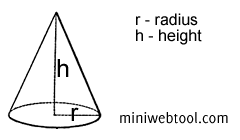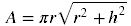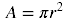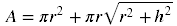×

# Surface Area of a Cone Calculator## About Surface Area of a Cone Calculator

The Surface Area of a Cone Calculator is used to help you find the surface area of a cone.

## Surface Area of a Cone Formula

The following is the calculation formula for surface area of a cone:

1. Lateral surface area of a cone2. Base surface area of a cone3. Total surface area of a coneWhere:
A = surface area of a cone
π = 3.141592654
h = height

## All of Our Miniwebtools (Sorted by Name):

•{{name}}
{{title}}

Miniwebtool

If you like Surface Area of a Cone Calculator please consider adding a link to this tool by copy/paste the following code:

Watch Videos: